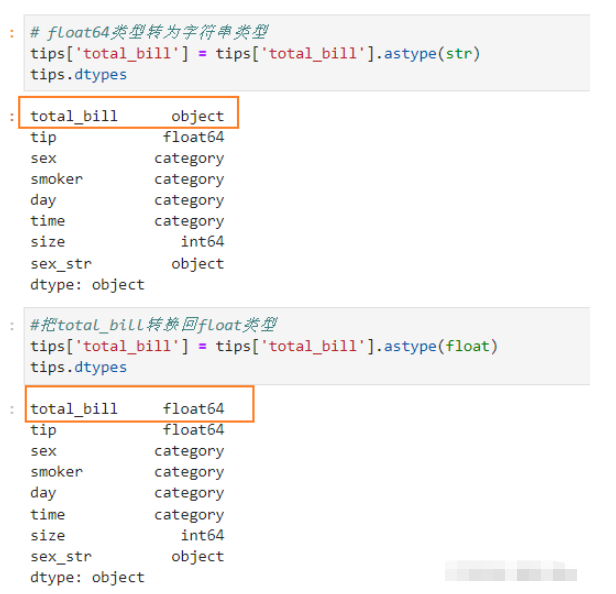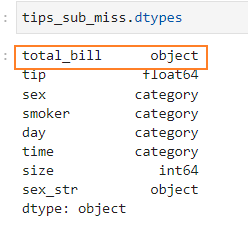# python数据处理之Pandas类型转换怎么实现

### 转换为字符串类型

`tips['sex_str'] = tips['sex'].astype(str)`### 转换为数值类型### 转为数值类型还可以使用to_numeric()函数

DataFrame每一列的数据类型必须相同，当有些数据中有缺失，但不是NaN时（如missing,null等），会使整列数据变成字符串类型而不是数值型，这个时候可以使用to_numeric处理

```#创造包含'missing'为缺失值的数据
tips_sub_miss.loc[[1,3,5,7],'total_bill'] = 'missing'
tips_sub_miss````tips_sub_miss['total_bill'].astype(float)``直接使用to_numeric()函数还是会报错，添加errors参数`

`errors`可变参数：

• `ignore` 遇到错误跳过 （只是跳过没转类型）

• `coerce` 遇到不能转的值强转为NaN

`pd.to_numeric(tips_sub_miss['total_bill'],errors='ignore')``pd.to_numeric(tips_sub_miss['total_bill'],errors='coerce')`to_numeric向下转型:

`downcast`参数

• `integer``signed`最小的有符号int dtype

• `float` 最小的float dtype

• `unsigned` 最小的无符号int dtype

downcast参数设置为float之后, total_bill的数据类型由float64变为float32

`pd.to_numeric(tips_sub_miss['total_bill'],errors='coerce',downcast='float')`### 分类数据（Category）

• 参1 values，如果values中的值，不在categories参数中，会被NaN代替

• 参2 categories，指定可能存在的类别数据

• 参3 ordered, 是否指定顺序

`s = pd.Series(pd.Categorical(["a","b","c","d"],categories=['c','b','a']))`ordered指定顺序：```from pandas.api.types import CategoricalDtype
# 创建一个分类  ordered  指定顺序
cat = CategoricalDtype(categories=['B','D','A','C'],ordered=True)
# 指定series_cat1转换类型为创建的分类类型
series_cat1 = series_cat.astype(cat)
print(series_cat.sort_values())
print(series_cat1.sort_values())```### 数据类型小结

Numpy的特点 1. Numpy是一个高效科学计算库，Pandas的数据计算功能是对Numpy的封装

2. ndarray是Numpy的基本数据结构，Pandas的Series和DataFrame好多函数和属性都与ndarray一样

3. Numpy的计算效率比原生Python效率高很多，并且支持并行计算
Pandas数据类型转换 1. Pandas除了数值型的int 和 float类型外，还有object ，category，bool，datetime类型

2. 可以通过as_type 和 to_numeric 函数进行数据类型转换
Pandas 分类数据类型 1. category类型，可以用来进行排序，并且可以自定义排序顺序

2. CategoricalDtype可以用来定义顺序